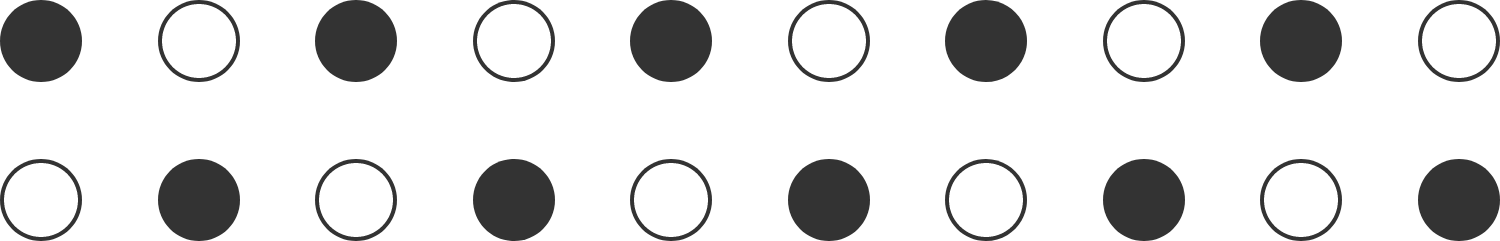# White Dot, Black Dot

Probability Level 4

The diagram below shows a $2 \times 10$ grid of dots (of negligible size) that alternate between black and white. How many ways are there to draw ten line segments between the dots such that

• each white dot is connected to exactly one black dot (and vice versa),
• no segment passes through more than two dots, and
• none of the segments intersect each other?Bonus: Generalize for drawing $n$ segments on a $2 \times n$ grid, where $n$ is a positive integer.

×